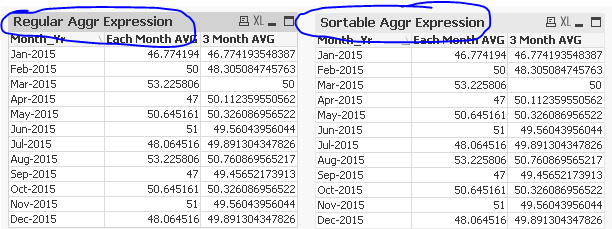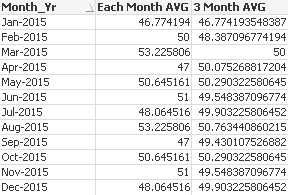# QlikView App Dev

Discussion Board for collaboration related to QlikView App Development.

Announcements
Our next Qlik Insider session will cover new key capabilities. Join us August 11th REGISTER TODAY
cancel
Showing results for
Did you mean:Partner

## 3 Months Average in Straight Table ?

Hi Techies,

I need to find average of 3 months in a  straight table. Here Month_Yr is dimension. I have measure field day wise from source table.

Example:

For Month_Yr "Mar-2015", calculate average for values from 01-Jan-2015 to 31-Mar-2015.

For Month_Yr "Apr-2015", calculate average for values from 01-Feb-2015 to 30-Apr-2015 and so on.

Here I have attached Test files for your reference.

1 Solution

Accepted SolutionsMVP

Script:

Sample:

Floor(MonthEnd(Date)) - MonthStart(Date, -2) + 1 as Days,

Measure,

Month(Date)&'-'&Year(Date) as Month_Yr

FROM

[Sample Data (1).xlsx]

(ooxml, embedded labels, table is Sheet1);

Where Days field will create a field which will help us know how many days do we need to go back for each month (which are not static). So months will have 91, 90, or 89 daysOnce you get this under your belt, the next step is to use the following formula, but this will only work in QV12 (because sorting in Aggr is only available in QV12 or Qlik Sense The sortable Aggr function is finally here!)

=Aggr(If(Date = Floor(MonthEnd(Date)), RangeAvg(Above(Avg(Measure), 0, Days))), (Date, (NUMERIC)))

If you don't have QV12, you will need to sort the date in the script, which might be difficult if you can bring your master calendar before anything else. If you can do this, then you can use this:

=Aggr(If(Date = Floor(MonthEnd(Date)), RangeAvg(Above(Avg(Measure), 0, Days))), Date)Regular expression works here because your data was well sorted in the Excel file, which might not be true for your real data.

HTH

Best,

Sunny

14 RepliesChampion IIPartner
Author

Hi Vineeth,

I need this in straight table without making changes in script.MVP

try this expression

=RangeAvg( above(avg(Measure),0,3))Partner
Author

Hi Kushal,

For example,

Month1 => 10, 20, 30, 40

Month2 => 50, 60, 70

Month3 => 90, 10, 20, 30

Month1=> (10+20+30+40)/4 => (100/4) => 25

Month2=> (50+60+70)/3 => (180/3) => 60

Month3=> (90+10+20+30)/4 => (150/4) => 37.5

then, (25+60+37.5)/3  => (122.5/3) = 40.8333...

But actually it should take average for 3 months with day wise values. Therefore  it should do,

(10+20+30+40+50+60+70+90+10+20+30) / 11 = 39.0909...

Here 39.0909 is the required result.

How can I achieve this.........MVP

I done the logic as per your result needed excel file which is matching with your needed outputMVP

what is the expected output you need for Mar - 2015?Partner
Author

Hi Kushal,

Required ResultValues after decimal point does not match... Because average has to be applied as per the above example.MVP

You want to show last months data while selection or Constant?

Before develop something, think If placed (The Right information | To the right people | At the Right time | In the Right place | With the Right context)MVP

Script:

Sample:

Floor(MonthEnd(Date)) - MonthStart(Date, -2) + 1 as Days,

Measure,

Month(Date)&'-'&Year(Date) as Month_Yr

FROM

[Sample Data (1).xlsx]

(ooxml, embedded labels, table is Sheet1);

Where Days field will create a field which will help us know how many days do we need to go back for each month (which are not static). So months will have 91, 90, or 89 daysOnce you get this under your belt, the next step is to use the following formula, but this will only work in QV12 (because sorting in Aggr is only available in QV12 or Qlik Sense The sortable Aggr function is finally here!)

=Aggr(If(Date = Floor(MonthEnd(Date)), RangeAvg(Above(Avg(Measure), 0, Days))), (Date, (NUMERIC)))

If you don't have QV12, you will need to sort the date in the script, which might be difficult if you can bring your master calendar before anything else. If you can do this, then you can use this:

=Aggr(If(Date = Floor(MonthEnd(Date)), RangeAvg(Above(Avg(Measure), 0, Days))), Date)Regular expression works here because your data was well sorted in the Excel file, which might not be true for your real data.

HTH

Best,

SunnyCommunity Browser